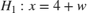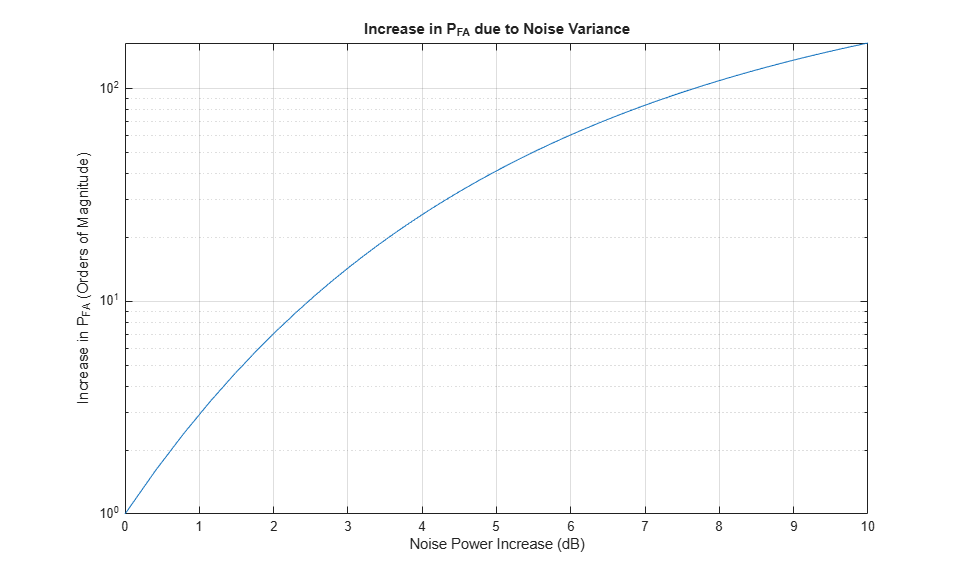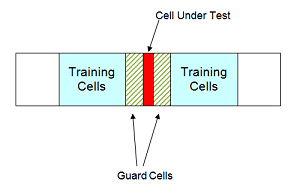## Constant False-Alarm Rate (CFAR) Detectors

### False Alarm Rate for CFAR Detectors

In the Neyman-Pearson framework, the probability of detection is maximized subject to the constraint that the false-alarm probability does not exceed a specified level. The false-alarm probability depends on the noise variance. Therefore, to calculate the false-alarm probability, you must first estimate the noise variance. If the noise variance changes, you must adjust the threshold to maintain a constant false-alarm rate. Constant false-alarm rate detectors implement adaptive procedures that enable you to update the threshold level of your test when the power of the interference changes.

To motivate the need for an adaptive procedure, assume a simple binary hypothesis test where you must decide between the signal-absent and signal-present hypotheses for a single sample. The signal has amplitude 4, and the noise is zero-mean Gaussian with unit variance.First, set the false-alarm rate to 0.001 and determine the threshold.

T = npwgnthresh(1e-3,1,'real'); threshold = sqrt(db2pow(T)) 
threshold = 3.0902 

Check that this threshold yields the desired false-alarm rate probability and then compute the probability of detection.

pfa = 0.5*erfc(threshold/sqrt(2)) pd = 0.5*erfc((threshold-4)/sqrt(2)) 
pfa = 1.0000e-03 pd = 0.8185 

Next, assume that the noise power increases by 6.02 dB, doubling the noise variance. If your detector does not adapt to this increase in variance by determining a new threshold, your false-alarm rate increases significantly.

pfa = 0.5*erfc(threshold/2) 
pfa = 0.0144 

The following figure shows the effect of increasing the noise variance on the false-alarm probability for a fixed threshold.

noisevar = 1:0.1:10; noisepower = 10*log10(noisevar); pfa = 0.5*erfc(threshold./sqrt(2*noisevar)); semilogy(noisepower,pfa./1e-3) grid on title('Increase in P_{FA} due to Noise Variance') ylabel('Increase in P_{FA} (Orders of Magnitude)') xlabel('Noise Power Increase (dB)')### Cell-Averaging CFAR Detector

The cell-averaging CFAR detector estimates the noise variance for the range cell of interest, or cell under test, by analyzing data from neighboring range cells designated as training cells. The noise characteristics in the training cells are assumed to be identical to the noise characteristics in the cell under test (CUT).

This assumption is key in justifying the use of the training cells to estimate the noise variance in the CUT. Additionally, the cell-averaging CFAR detector assumes that the training cells do not contain any signals from targets. Thus, the data in the training cells are assumed to consist of noise only.

To make these assumptions realistic:

• It is preferable to have some buffer, or guard cells, between the CUT and the training cells. The buffer provided by the guard cells guards against signal leaking into the training cells and adversely affecting the estimation of the noise variance.

• The training cells should not represent range cells too distant in range from the CUT, as the following figure illustrates.The optimum estimator for the noise variance depends on distributional assumptions and the type of detector. Assume the following:

1. You are using a square-law detector.

2. You have a Gaussian, complex-valued, random variable (RV) with independent real and imaginary parts.

3. The real and imaginary parts each have mean zero and variance equal to σ2/2.

Note

If you denote this RV by Z=U+jV, the squared magnitude |Z|2 follows an exponential distribution with mean σ2.

If the samples in training cells are the squared magnitudes of such complex Gaussian RVs, you can use the sample mean as an estimator of the noise variance.

To implement cell-averaging CFAR detection, use phased.CFARDetector. You can customize characteristics of the detector such as the numbers of training cells and guard cells, and the probability of false alarm.

### CFAR Detector Adaptation to Noisy Input Data

This example shows how to create a CFAR detector and test its ability to adapt to the statistics of input data. The test uses noise-only trials. By using the default square-law detector, you can determine how close the empirical false-alarm rate is to the desired false-alarm probability.

Note: This example runs only in R2016b or later. If you are using an earlier release, replace each call to the function with the equivalent step syntax. For example, replace myObject(x) with step(myObject,x).

Create a CFAR detector object with two guard cells, 20 training cells, and a false-alarm probability of 0.001. By default, this object assumes a square-law detector with no pulse integration.

detector = phased.CFARDetector('NumGuardCells',2,... 'NumTrainingCells',20,'ProbabilityFalseAlarm',1e-3);

There are 10 training cells and 1 guard cell on each side of the cell under test (CUT). Set the CUT index to 12.

CUTidx = 12;

Seed the random number generator for a reproducible set of input data.

rng(1000);

Set the noise variance to 0.25. This value corresponds to an approximate -6 dB SNR. Generate a 23-by-10000 matrix of complex-valued, white Gaussian rv's with the specified variance. Each row of the matrix represents 10,000 Monte Carlo trials for a single cell.

Ntrials = 1e4; variance = 0.25; Ncells = 23; inputdata = sqrt(variance/2)*(randn(Ncells,Ntrials)+1j*randn(Ncells,Ntrials));

Because the example implements a square-law detector, take the squared magnitudes of the elements in the data matrix.

Z = abs(inputdata).^2;

Provide the output from the square-law operator and the index of the cell under test to the CFAR detector.

Z_detect = detector(Z,CUTidx);

The output, Z_detect, is a logical vector with 10,000 elements. Sum the elements in Z_detect and divide by the total number of trials to obtain the empirical false-alarm rate.

pfa = sum(Z_detect)/Ntrials
pfa = 0.0013 

The empirical false-alarm rate is 0.0013, which corresponds closely to the desired false-alarm rate of 0.001.

### Extensions of Cell-Averaging CFAR Detector

The cell-averaging algorithm for a CFAR detector works well in many situations, but not all. For example, when targets are closely located, cell averaging can cause a strong target to mask a weak target nearby. The phased.CFARDetector System object™ supports the following CFAR detection algorithms.

AlgorithmTypical Usage
Cell-averaging CFARMost situations
Greatest-of cell-averaging CFARWhen it is important to avoid false alarms at the edge of clutter
Smallest-of cell-averaging CFARWhen targets are closely located
Order statistic CFARCompromise between greatest-of and smallest-of cell averaging

### Detection Probability for CFAR Detector

This example shows how to compare the probability of detection resulting from two CFAR algorithms. In this scenario, the order statistic algorithm detects a target that the cell-averaging algorithm does not.

Note: This example runs only in R2016b or later. If you are using an earlier release, replace each call to the function with the equivalent step syntax. For example, replace myObject(x) with step(myObject,x).

Create a CFAR detector that uses the cell-averaging CFAR algorithm.

Ntraining = 10; Nguard = 2; Pfa_goal = 0.01; detector = phased.CFARDetector('Method','CA',... 'NumTrainingCells',Ntraining,'NumGuardCells',Nguard,... 'ProbabilityFalseAlarm',Pfa_goal);

The detector has 2 guard cells, 10 training cells, and a false-alarm probability of 0.01. This object assumes a square-law detector with no pulse integration.

Generate a vector of input data based on a complex-valued white Gaussian random variable.

Ncells = 23; Ntrials = 100000; inputdata = 1/sqrt(2)*(randn(Ncells,Ntrials) + ... 1i*randn(Ncells,Ntrials));

In the input data, replace rows 8 and 12 to simulate two targets for the CFAR detector to detect.

inputdata(8,:) = 3*exp(1i*2*pi*rand); inputdata(12,:) = 9*exp(1i*2*pi*rand);

Because the example implements a square-law detector, take the squared magnitudes of the elements in the input data vector.

Z = abs(inputdata).^2;

Perform the detection on rows 8 through 12.

Z_detect = detector(Z,8:12);

The Z_detect matrix has five rows. The first and last rows correspond to the simulated targets. The three middle rows correspond to noise.

Compute the probability of detection of the two targets. Also, estimate the probability of false alarm using the noise-only rows.

Pd_1 = sum(Z_detect(1,:))/Ntrials
Pd_1 = 0 
Pd_2 = sum(Z_detect(end,:))/Ntrials
Pd_2 = 1 
Pfa = max(sum(Z_detect(2:end-1,:),2)/Ntrials)
Pfa = 6.0000e-05 

The 0 value of Pd_1 indicates that this detector does not detect the first target.

Change the CFAR detector so it uses the order statistic CFAR algorithm with a rank of 5.

release(detector); detector.Method = 'OS'; detector.Rank = 5;

Repeat the detection and probability computations.

Z_detect = detector(Z,8:12); Pd_1 = sum(Z_detect(1,:))/Ntrials
Pd_1 = 0.5820 
Pd_2 = sum(Z_detect(end,:))/Ntrials
Pd_2 = 1 
Pfa = max(sum(Z_detect(2:end-1,:),2)/Ntrials)
Pfa = 0.0066 

Using the order statistic algorithm instead of the cell-averaging algorithm, the detector detects the first target in about 58% of the trials.